# Chapter 13 chemical reactions

1 de Mar de 2015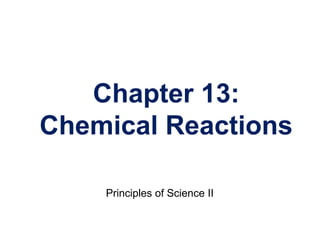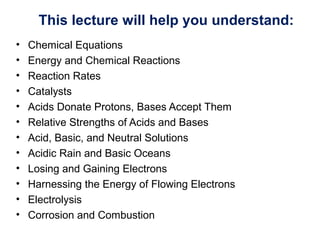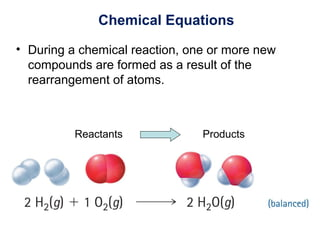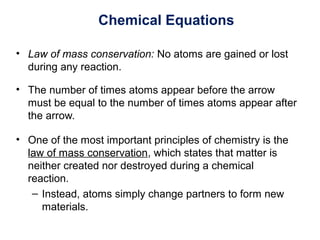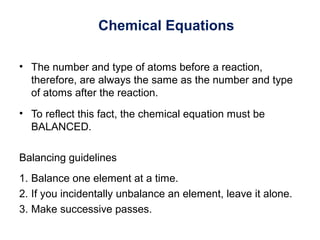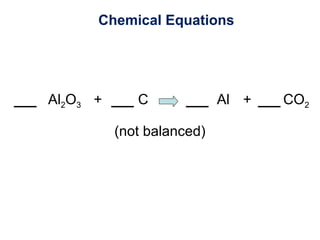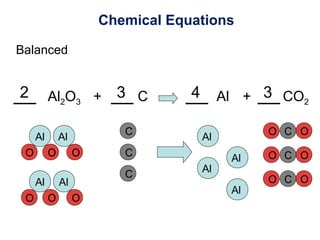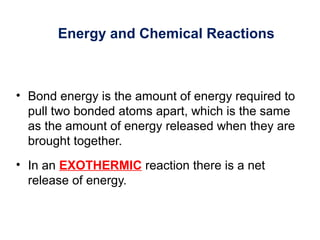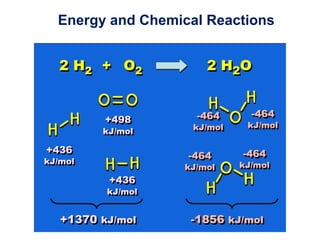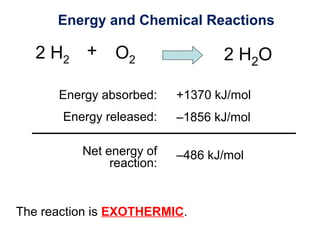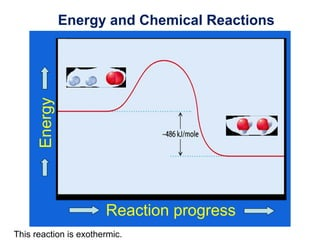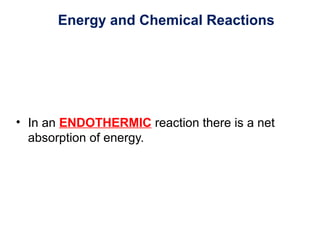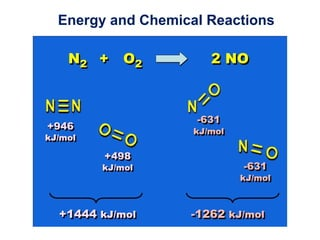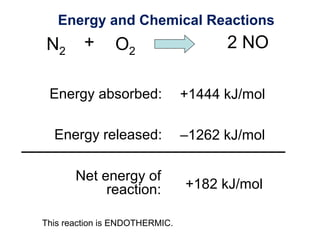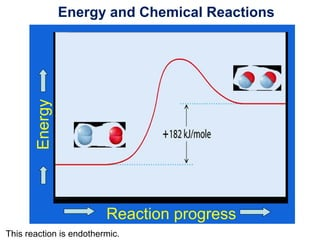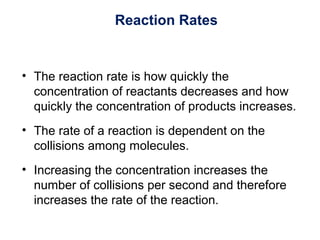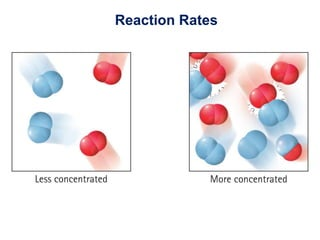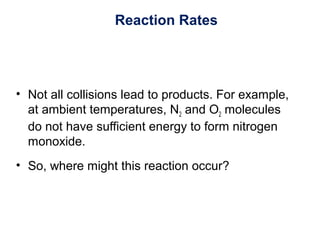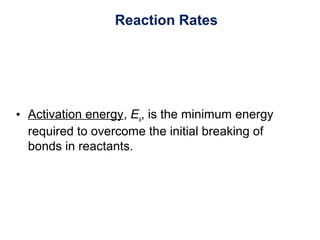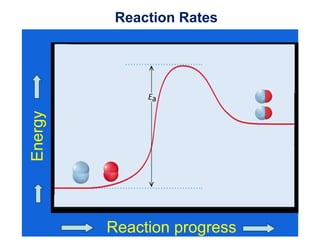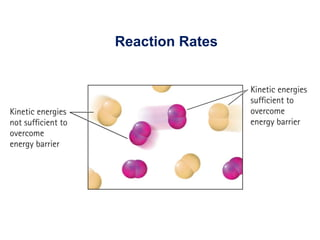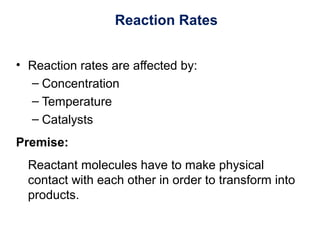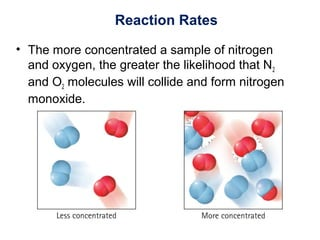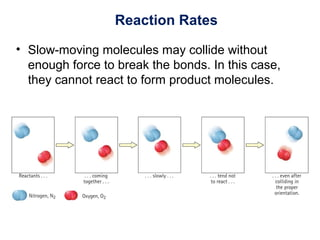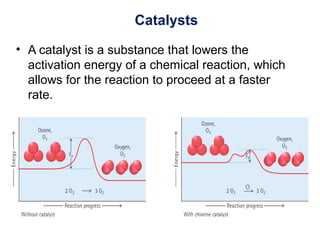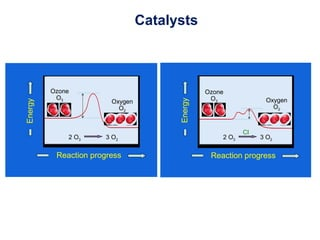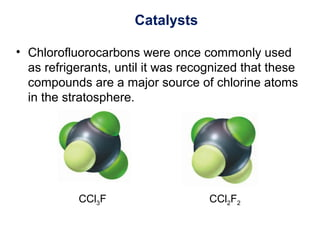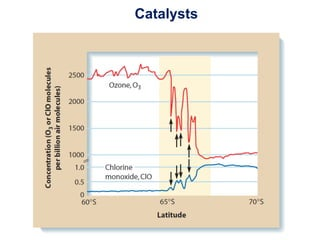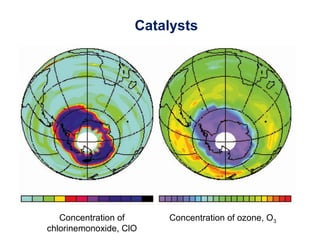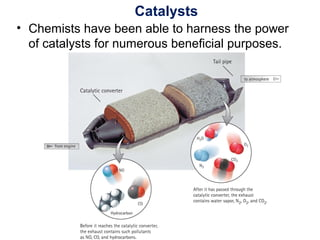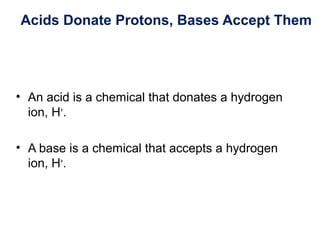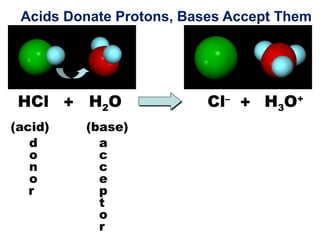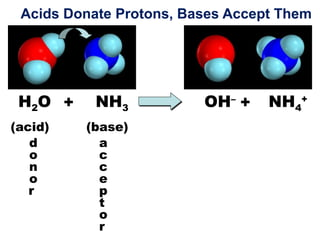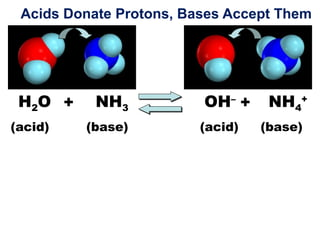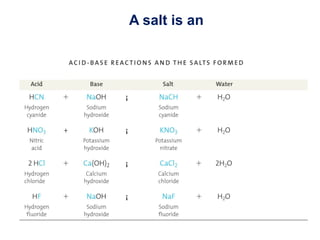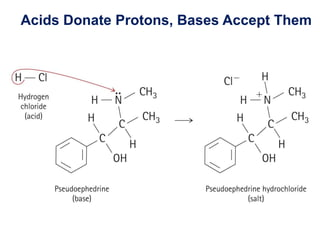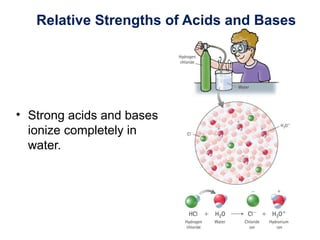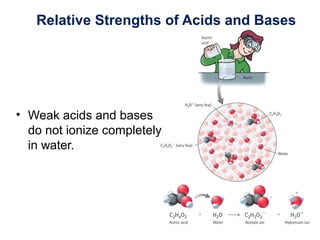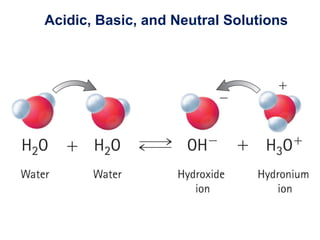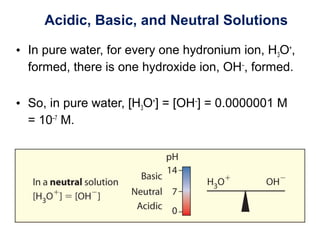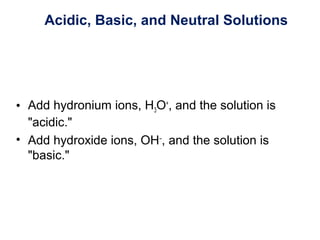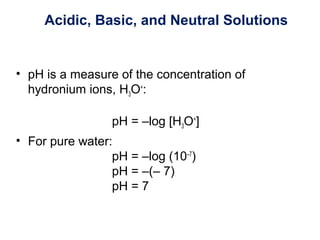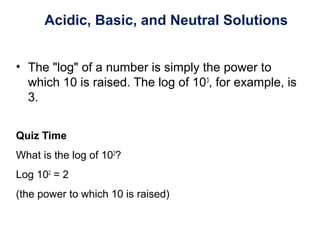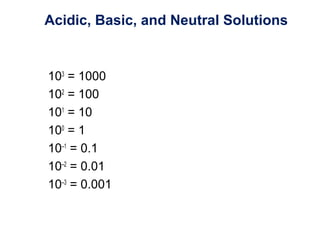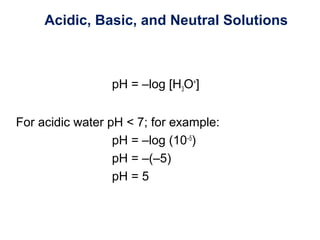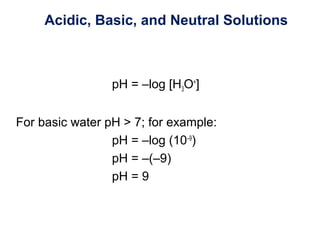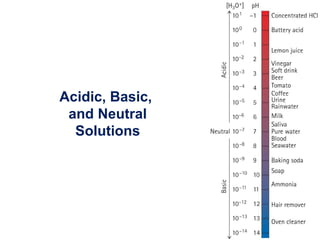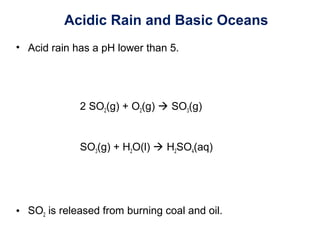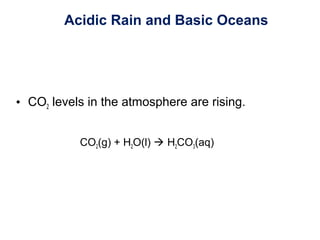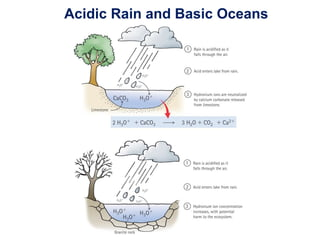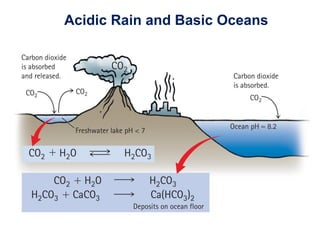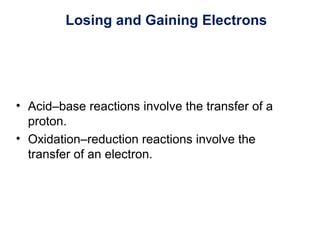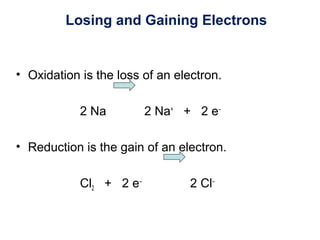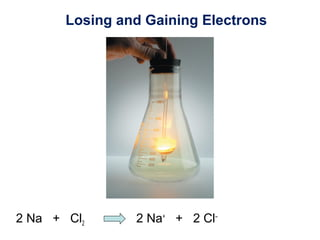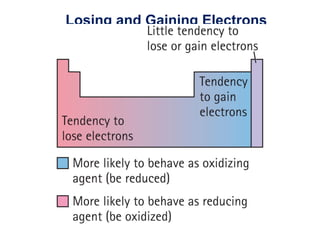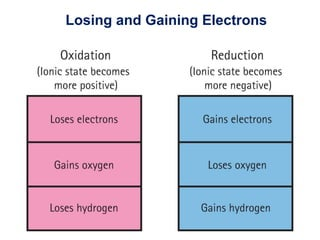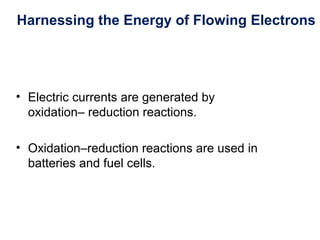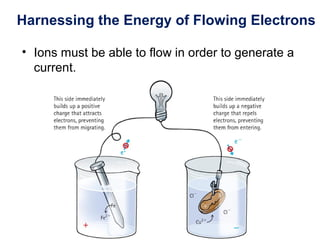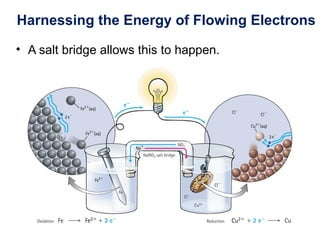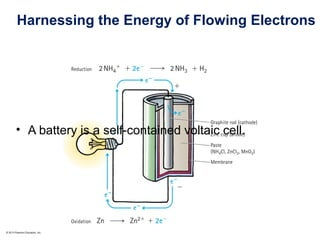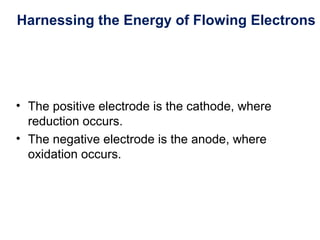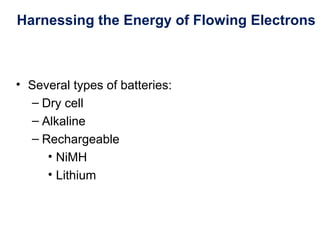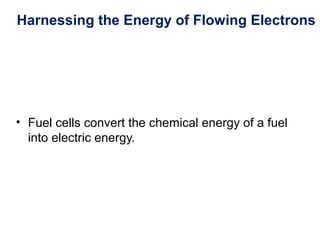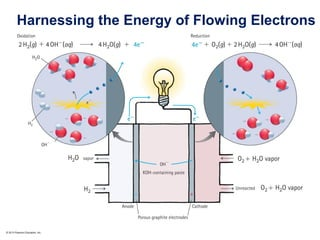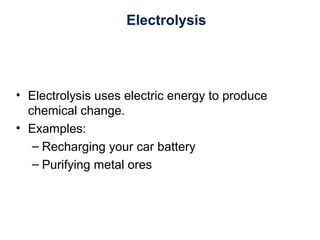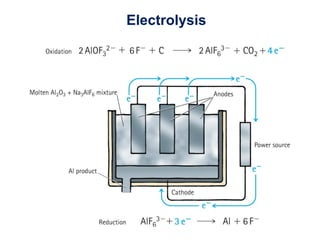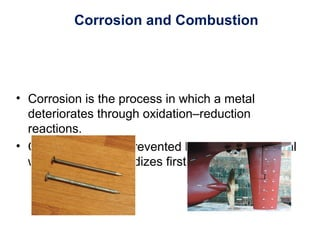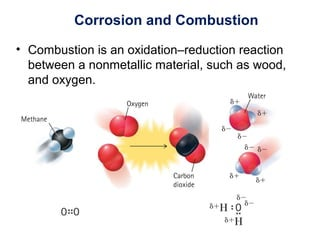1 de 69

### Chapter 13 chemical reactions

• 2. This lecture will help you understand: • Chemical Equations • Energy and Chemical Reactions • Reaction Rates • Catalysts • Acids Donate Protons, Bases Accept Them • Relative Strengths of Acids and Bases • Acid, Basic, and Neutral Solutions • Acidic Rain and Basic Oceans • Losing and Gaining Electrons • Harnessing the Energy of Flowing Electrons • Electrolysis • Corrosion and Combustion
• 3. Reactants Products Chemical Equations • During a chemical reaction, one or more new compounds are formed as a result of the rearrangement of atoms.
• 4. Chemical Equations • Law of mass conservation: No atoms are gained or lost during any reaction. • The number of times atoms appear before the arrow must be equal to the number of times atoms appear after the arrow. • One of the most important principles of chemistry is the law of mass conservation, which states that matter is neither created nor destroyed during a chemical reaction. – Instead, atoms simply change partners to form new materials.
• 5. Chemical Equations • The number and type of atoms before a reaction, therefore, are always the same as the number and type of atoms after the reaction. • To reflect this fact, the chemical equation must be BALANCED. Balancing guidelines 1. Balance one element at a time. 2. If you incidentally unbalance an element, leave it alone. 3. Make successive passes.
• 6. Al2O3 C CO2+ Al + (not balanced) Chemical Equations
• 7. 4 32 3 Al Al OOO C Al C OO Al C OO C OOAl Al OOO C C Al Al Al2O3 C CO2+ Al + Chemical Equations Balanced
• 8. Energy and Chemical Reactions • Bond energy is the amount of energy required to pull two bonded atoms apart, which is the same as the amount of energy released when they are brought together. • In an EXOTHERMIC reaction there is a net release of energy.
• 10. 2 H2 O2 2 H2O+ Energy and Chemical Reactions The reaction is EXOTHERMIC. Energy absorbed: Energy released: –486 kJ/molNet energy of reaction: –1856 kJ/mol +1370 kJ/mol
• 11. Energy and Chemical Reactions Reaction progress Energy This reaction is exothermic.
• 12. Energy and Chemical Reactions • In an ENDOTHERMIC reaction there is a net absorption of energy.
• 13. Energy and Chemical Reactions
• 14. +1444 kJ/mol –1262 kJ/mol Energy absorbed: Energy released: Net energy of reaction: N2 O2 2 NO+ Energy and Chemical Reactions +182 kJ/mol This reaction is ENDOTHERMIC.
• 15. Energy and Chemical Reactions Reaction progress Energy This reaction is endothermic.
• 16. Reaction Rates • The reaction rate is how quickly the concentration of reactants decreases and how quickly the concentration of products increases. • The rate of a reaction is dependent on the collisions among molecules. • Increasing the concentration increases the number of collisions per second and therefore increases the rate of the reaction.
• 18. Reaction Rates • Not all collisions lead to products. For example, at ambient temperatures, N2 and O2 molecules do not have sufficient energy to form nitrogen monoxide. • So, where might this reaction occur?
• 19. Reaction Rates • Activation energy, Ea, is the minimum energy required to overcome the initial breaking of bonds in reactants.
• 21. Reaction Rates • Reactants must be moving fast enough (have sufficient kinetic energy) to overcome the energy of activation.
• 22. Reaction Rates • Reaction rates are affected by: – Concentration – Temperature – Catalysts Premise: Reactant molecules have to make physical contact with each other in order to transform into products.
• 23. Reaction Rates • The more concentrated a sample of nitrogen and oxygen, the greater the likelihood that N2 and O2 molecules will collide and form nitrogen monoxide.
• 24. Reaction Rates • Slow-moving molecules may collide without enough force to break the bonds. In this case, they cannot react to form product molecules.
• 25. Catalysts • A catalyst is a substance that lowers the activation energy of a chemical reaction, which allows for the reaction to proceed at a faster rate.
• 26. Reaction progress Energy Ozone O3 Oxygen O2 2 O3 3 O2 Cl Reaction progress Energy Ozone O3 Oxygen O2 2 O3 3 O2 Catalysts
• 27. CCl3F CCl2F2 Catalysts • Chlorofluorocarbons were once commonly used as refrigerants, until it was recognized that these compounds are a major source of chlorine atoms in the stratosphere.
• 30. Catalysts • Chemists have been able to harness the power of catalysts for numerous beneficial purposes.
• 31. Acids Donate Protons, Bases Accept Them • An acid is a chemical that donates a hydrogen ion, H+ . • A base is a chemical that accepts a hydrogen ion, H+ .
• 32. H3O+ Cl– H2OHCl + + Acids Donate Protons, Bases Accept Them (acid) (base) d o n o r a c c e p t o r
• 33. NH4 + OH– +H2O NH3 d o n o r a c c e p t o r + Acids Donate Protons, Bases Accept Them (acid) (base)
• 34. NH4 + OH– +H2O NH3+ Acids Donate Protons, Bases Accept Them (acid) (base) (acid) (base)
• 35. A salt is an
• 36. Acids Donate Protons, Bases Accept Them
• 37. Relative Strengths of Acids and Bases • Strong acids and bases ionize completely in water.
• 38. Relative Strengths of Acids and Bases • Weak acids and bases do not ionize completely in water.
• 39. Acidic, Basic, and Neutral Solutions • Water can behave as an acid or a base.
• 40. Acidic, Basic, and Neutral Solutions • In pure water, for every one hydronium ion, H3O+ , formed, there is one hydroxide ion, OH– , formed. • So, in pure water, [H3O+ ] = [OH– ] = 0.0000001 M = 10–7 M.
• 41. Acidic, Basic, and Neutral Solutions • Add hydronium ions, H3O+ , and the solution is "acidic." • Add hydroxide ions, OH– , and the solution is "basic."
• 42. © 2013 Pearson Education, Inc. Acidic, Basic, and Neutral Solutions
• 43. Acidic, Basic, and Neutral Solutions • pH is a measure of the concentration of hydronium ions, H3O+ : pH = –log [H3O+ ] • For pure water: pH = –log (10–7 ) pH = –(– 7) pH = 7
• 44. Acidic, Basic, and Neutral Solutions • The "log" of a number is simply the power to which 10 is raised. The log of 103 , for example, is 3. Quiz Time What is the log of 102 ? Log 102 = 2 (the power to which 10 is raised)
• 45. Acidic, Basic, and Neutral Solutions 103 = 1000 102 = 100 101 = 10 100 = 1 10–1 = 0.1 10–2 = 0.01 10–3 = 0.001
• 46. Acidic, Basic, and Neutral Solutions pH = –log [H3O+ ] For acidic water pH < 7; for example: pH = –log (10–5 ) pH = –(–5) pH = 5
• 47. Acidic, Basic, and Neutral Solutions pH = –log [H3O+ ] For basic water pH > 7; for example: pH = –log (10–9 ) pH = –(–9) pH = 9
• 49. Acidic Rain and Basic Oceans • Acid rain has a pH lower than 5. 2 SO2(g) + O2(g)  SO3(g) SO3(g) + H2O(l)  H2SO4(aq) • SO2 is released from burning coal and oil.
• 50. Acidic Rain and Basic Oceans • CO2 levels in the atmosphere are rising. CO2(g) + H2O(l)  H2CO3(aq)
• 51. Acidic Rain and Basic Oceans
• 52. Acidic Rain and Basic Oceans
• 53. Losing and Gaining Electrons • Acid–base reactions involve the transfer of a proton. • Oxidation–reduction reactions involve the transfer of an electron.
• 54. Losing and Gaining Electrons • Oxidation is the loss of an electron. 2 Na 2 Na+ + 2 e– • Reduction is the gain of an electron. Cl2 + 2 e– 2 Cl–
• 55. Losing and Gaining Electrons 2 Na + Cl2 2 Na+ + 2 Cl–
• 56. Losing and Gaining Electrons
• 57. Losing and Gaining Electrons
• 58. Harnessing the Energy of Flowing Electrons • Electric currents are generated by oxidation– reduction reactions. • Oxidation–reduction reactions are used in batteries and fuel cells.
• 59. Harnessing the Energy of Flowing Electrons • Ions must be able to flow in order to generate a current.
• 60. Harnessing the Energy of Flowing Electrons • A salt bridge allows this to happen.
• 61. © 2013 Pearson Education, Inc. Harnessing the Energy of Flowing Electrons • A battery is a self-contained voltaic cell.
• 62. Harnessing the Energy of Flowing Electrons • The positive electrode is the cathode, where reduction occurs. • The negative electrode is the anode, where oxidation occurs.
• 63. Harnessing the Energy of Flowing Electrons • Several types of batteries: – Dry cell – Alkaline – Rechargeable • NiMH • Lithium
• 64. Harnessing the Energy of Flowing Electrons • Fuel cells convert the chemical energy of a fuel into electric energy.
• 65. © 2013 Pearson Education, Inc. Harnessing the Energy of Flowing Electrons
• 66. Electrolysis • Electrolysis uses electric energy to produce chemical change. • Examples: – Recharging your car battery – Purifying metal ores
• 68. Corrosion and Combustion • Corrosion is the process in which a metal deteriorates through oxidation–reduction reactions. • Corrosion can be prevented by coating the metal with zinc, which oxidizes first.
• 69. Corrosion and Combustion • Combustion is an oxidation–reduction reaction between a nonmetallic material, such as wood, and oxygen.